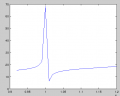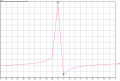# Is there a way to swap the location of resonance and anti-resonance?

#### tx.x

Joined Jun 27, 2017
14

#### Alec_t

Joined Sep 17, 2013
12,315
Try swapping L for C and C for L.

#### BR-549

Joined Sep 22, 2013
4,938
It's a very open question.....just showing a network. Do you have any practical context? A circuit or a function that your working on?

Have you tried flipping the circuit over?

Edit: Sorry Alec t.......didn't see your post.

#### tx.x

Joined Jun 27, 2017
14
It's a very open question.....just showing a network. Do you have any practical context? A circuit or a function that your working on?

Have you tried flipping the circuit over?

Edit: Sorry Alec t.......didn't see your post.
Yes I have, didn't work

#### tx.x

Joined Jun 27, 2017
14

#### Ylli

Joined Nov 13, 2015
1,053
Pretty sure you are going to want to replace the shunt C with a shunt L. Reactance of the L about the same as that of the removed C.

Currently you have a series resonant circuit. Below resonance, the series section look like a capacitor - and adding the shunt C only makes it more C. Above resonance, the series branch looks like an inductance, and the shunt C creates a parallel resonance.

You want to reverse that. You still want the series branch to be resonant where it is, and know it will look capacitive below resonance. You want to resonate that capacitance with a parallel L.

#### tx.x

Joined Jun 27, 2017
14
Thanks for your reply... but I believe you are plotting the reactance in dB ....while I'm plotting it in Ohm...

#### tx.x

Joined Jun 27, 2017
14
hmm..now I'm confused..I verified using circuit simulator and formula and just couldn't seem to get your response.....

Here is the script I used:
>> C1=100e-12;
>> L1=250e-6;
>> L2=2.533e-6;
>> f=0.92e6:0.01e6:1.2e6;
>> w=2.*pi.*f;
>> z1=1j.*w.*L1+1./(1j.*w.*C1);
>> z2=1j.*w.*L2;
>> z_total=1./(1./z1+1./z2);
>> plot(imag(z_total))
>> plot(f, imag(z_total))

Here is the reactance in Ohm:Here is the response from simulation:#### Ylli

Joined Nov 13, 2015
1,053
Don't know what you are using to do these plots, but try using more frequencies. You've got a fairly high Q network with rapid changes in impedance. Checking it only every 0.01 MHz may not be enough.

As I say, I don't know what you are using to do the plotting, but your original plot seems wrong. You have a basic series resonant circuit, which will have a low impedance at resonance. A series resonant network will look like an inductance above it's resonant frequency and C2 will parallel resonate with that inductance. So the impedance plot *should* have an impedance minimum at a lower frequency that the impedance maximum.

That series resonant network will look like a capacitance below resonance. By replacing C2 with L2, you are now resonating that apparent C with L2 producing a parallel resonant circuit -below- the series resonant frequency.

Look closer in with you plotting app, use more frequencies.

#### tx.x

Joined Jun 27, 2017
14
Don't know what you are using to do these plots, but try using more frequencies. You've got a fairly high Q network with rapid changes in impedance. Checking it only every 0.01 MHz may not be enough.

As I say, I don't know what you are using to do the plotting, but your original plot seems wrong. You have a basic series resonant circuit, which will have a low impedance at resonance. A series resonant network will look like an inductance above it's resonant frequency and C2 will parallel resonate with that inductance. So the impedance plot *should* have an impedance minimum at a lower frequency that the impedance maximum.

That series resonant network will look like a capacitance below resonance. By replacing C2 with L2, you are now resonating that apparent C with L2 producing a parallel resonant circuit -below- the series resonant frequency.

Look closer in with you plotting app, use more frequencies.
I don't think you are right, the series resonant network will look like a capacitor below resonance and inductor above resonance, locally at the resonant frequency, the reactance is almost a straight line. Left side <0, crosses 0, then right side > 0.

Now I look at the susceptance, on the left side of resonance, it will be positive (1./1j = positive), continue to increase until resonance, then drop to a large negative value, then increase again.

Now I add an inductor in parallel with my series resonant LC circuit, the susceptance of the parallel inductor can also be modelled locally as a negative straight line approaching 0 from the negative side. I don't think it's possible to change the locations of resonance and anti-resonance this way.

I'm not sure what you were plotting but I'm pretty confident that I didn't do anything wrong, I'm also positive that it wasn't a problem with the plotting resolution (tried with smaller ones).